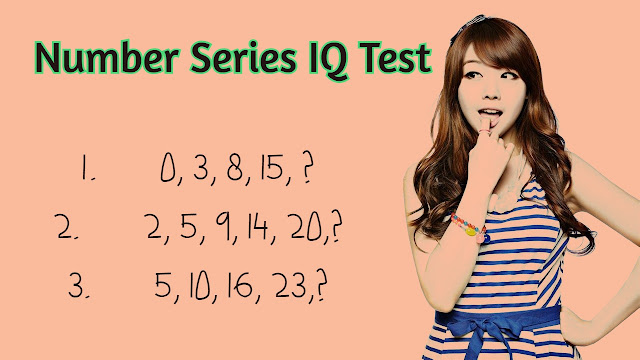20 Important Number Series IQ Question and Answer (Basic Number Series IQ Question) - It is very important those participants who participate in the competitive exam and normally other people also learn about the number series IQ. In Number Series IQ, there are given some series of numbers that are followed a similar pattern and ask you to find out the missing number of this series?

First of all, you considered the pattern of series which is followed by the previous number. There are probably given two or three-way pattern is follow but you find the correct pattern of given Number Series. The following some of the questions are given, first, you try the series question and the last check the answer is below sector. Ok, my dear friends, let's get started.Number Series IQ

1.       0, 3, 8, 15, ?
2.       2, 5, 9, 14, 20,?
3.       5, 10, 16, 23,?
4.      10, 13, 18, 25,?
5.      2, 3, 5, 8, 12,?
6.      7, 11, 13, 17, 19,?
7.       2, 8, 14, 20,?
8.       10, 17, 26, 37,?
9.       3, 8, 15, 24, 35,?
10.     20, 30, 42, 56,?
11.      1, 4, 9, 16, 25, 36,?
12.      1, 8, 27, 64, 125,?
13.      2, 3, 5, 8, 12,?
14.      1, 2, 6, 24,?
15.      1, 5, 20, 60,?
16.      5, 25, 125,?
17.     2, 5, 10, 17,?
18.      3, 4, 6, 9,?
19.      2, 4, 8, 16, 32,?
20.      2, 5, 11, 23,?

Check the Solution:

1. 0+3 = 3
3+5 = 8
8+7 = 15
15+9 = 24

Therefore, the correct answer is 24. This series are followed the increasing pattern by 2 form every previous elements i.e. (3, 5, 7, 9).

2. 2+3   = 5
5+4   = 9
9+5   = 14
14+6  = 20
20+7  = 27

Therefore, the correct answer is 27. This series are followed the increasing pattern by additional 1 from every previous elements i.e. (3, 4, 5, 6, 7, 8).

3. 5+5 = 10
10+6 = 16
16+7 = 23
23+8 = 31

Hence the correct answer is 31. This series are followed the increasing pattern by additional 1 from every previous elements i.e. (5, 6, 7, 8).

4. 10+3 = 13
13+5 = 18
18+7 = 25
25+9 = 34

Therefore, the correct answer is 26. This series are followed the increasing pattern by 2 from every previous elements i.e. (3,5,7,9).

5. 2+1 = 3
3+2 = 5
5+3 = 8
8+4 = 12
12+5 = 17

So that the correct answer is 17.This series are followed the increasing pattern by 1 form every previous elements i.e. (1, 2, 3, 4, 5).

6. 7+4  = 11
11+2 = 13
13+4 = 17
17+2 = 19
19+4 = 23

Therefore, the correct answer is 23. This series followed the first adding 4 and second declining by 2 every interval.

7. 2+6 = 8
8+6 = 14
14+6 = 20
20+6 = 26

Therefore, the correct answer is 26.This number series followed adding by 6 for every number of series.

8. 3*3+1 = 10
4*4+1 = 17
5*5+1 = 26
6*6+1 = 37
7*7+1 = 50

Therefore, the correct answer is 50. This series follow the squaring every regular number and adding by 1 for all number of series.

9. 3+5 = 8
8+7 = 15
15+9 = 24
24+11 = 35
35+13 = 48

Therefore, the correct answer is 48. This series are followed the increasing pattern by 2 from every previous elements i.e. (5, 7, 9, 11, 13,...).

10. 20+10 = 30
30+12 = 42
42+14 = 56
56+16 = 72

Therefore, the correct answer is 72. This series are followed the increasing pattern by 2 form every previous elements i.e. (10, 12, 14, 16,....).

11. 1*1 = 1
2*2 = 4
3*3 = 9
4*4 = 16
5*5 = 25
6*6 = 36
7*7 = 49

So that correct answer is 49. These series follow the squaring all number of given series.

12. 1*1*1 = 1
2*2*2 = 8
3*3*3 = 27
4*4*4 = 64
5*5*5 = 125
6*6*6 = 216

So that correct answer is 216. These every numbers are multiplied by additional 1 from previous increment.

13. 2+1 = 3
3+2 = 5
5+3 = 8
8+4 = 12
12+5 = 17

So that correct answer is 17. These every numbers are increased by additional 1 from previous increment.

14. 1*2 = 2
2*3 = 6
6*4 = 24
24*5 = 120

So that correct answer is 120.These every numbers are multiplied by additional 1 from previous increment.

15. 1*5 = 5
5*4 = 20
20*3 = 60
60*2 = 120

So that correct answer is 120.These every numbers are multiplied by additional 1 from previous increase.

16. 5*5 = 25
25*5 = 125
125*5 = 625

So that correct answer is 625. The given every element multiple by 5.

17. 2+3 = 5
5+5 = 10
10+7 = 17
17+9 = 26

So that correct answer is 26. This pattern of every numbers is increased by additional 2 from previous increment.

18. 3+1 = 4
4+2 = 6
6+3 = 9
9+4 = 13

So that correct answer is 13. These every numbers are increased by additional 1 from previous increment.

19. 2*2 = 4
4*2 = 8
8*2 = 16
16*2 = 32
32*2 = 64

So that correct answer is 64. Every elements are multiple by 2 for series.

20. 2*2+1 = 5
5*2+1 = 11
11*2+1 = 23
23*2+1 = 47

So that correct answer is 47. Every elements are multiple by 2 and adding 1 for all given series.

I hope, it is very useful for learning some tips and tricks about Number Series IQ. From related to this topic any question you have easily asked in below comment section. I will give an answer as soon as possible about your number series IQ related question.